# Scientific Calculator (adfree) v3.9.8 Apk

TechCalc+ contains 12 computing modes in one application + a handy reference section. Modes included are: * Basic Mode, * Scientific ...

TechCalc+ contains 12 computing modes in one application + a handy reference section.
Modes included are:
* Basic Mode,
* Scientific Mode,
* 64-bit Programmer Mode (Hex, Oct, Bin and Dec),
* Graphing (save copy of graph to sdcard),
* Matrices,
* Complex Numbers (cartesian, polar, using Euler's identity),
* Quick Formulas,
* Quick Converter,
* Time Calculator,
* Equation Solver,
* Calculus (Derivative, Definite Integral, Taylor Series & Indefinite Integral) - requires Android version 2.3 or higher
* Financial
+ the Periodic Table of Elements!
Features include:
* Powers & Roots
* Logs and Antilogs
* Factorial, Modulus & Random Numbers functions
* HCF, LCM, Prime factorization
* Pol() & Rec() Functions
* Permutations (nPr) and Combinations (nCr)
* Statistics
* Fractions Mode
* A wide range of conversion categories and constants
* 20 Memory Registers in each of the calculation modes
* Detailed calculation history
* Extensive Help and Reference
* Highly customizable via the Settings
The reference section includes:
* Physical Laws
* Names in the Metric System
* Mathematical Tables
* Elementary & Linear Algebra
* Trigonometric Identities
* Differentiation & Integration Rules
* Statistics Formulas
* Vector Mathematics
* ASCII, Fractional Bits, Roman Numeral & Number Base Converters
* pH, Interpolation, Body Mass Index (BMI), Percentage, Proportion & Molecular Weight Calculators
* Sigma & Pi Notation
* Balancing Chemical Equations
Please email any questions that are not answered in the Help section.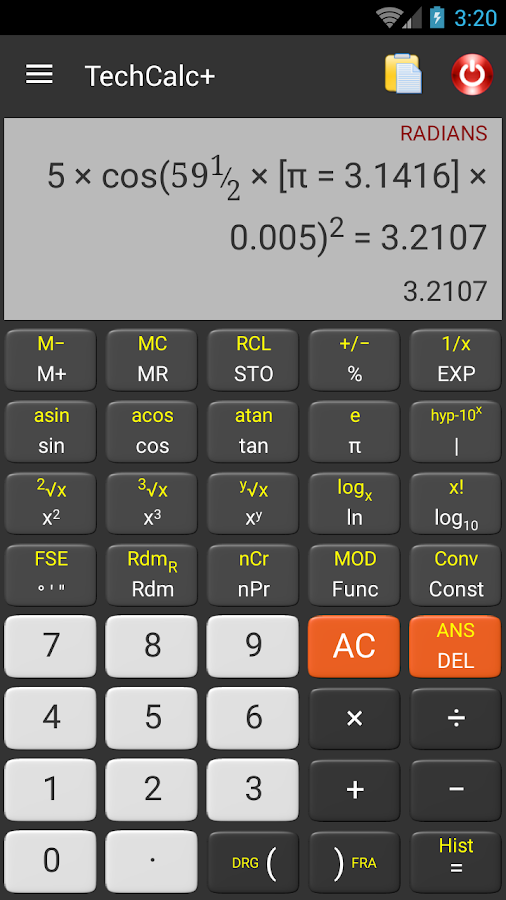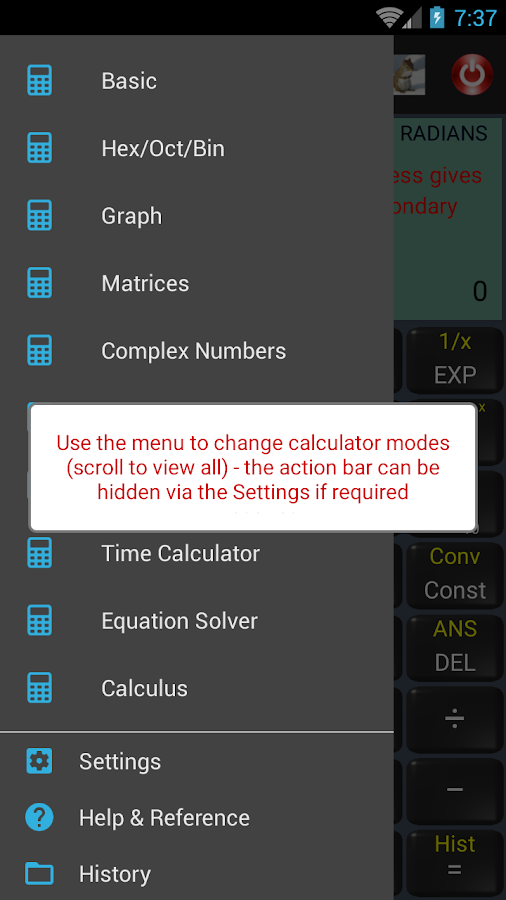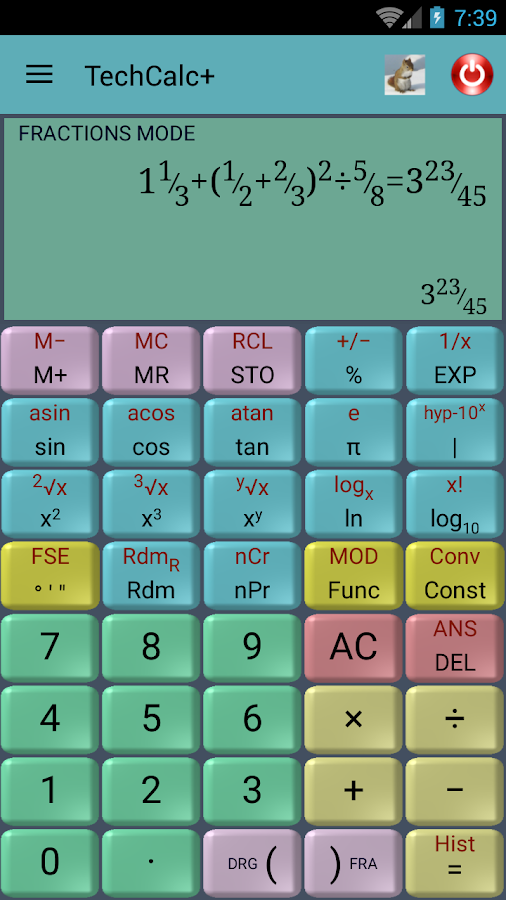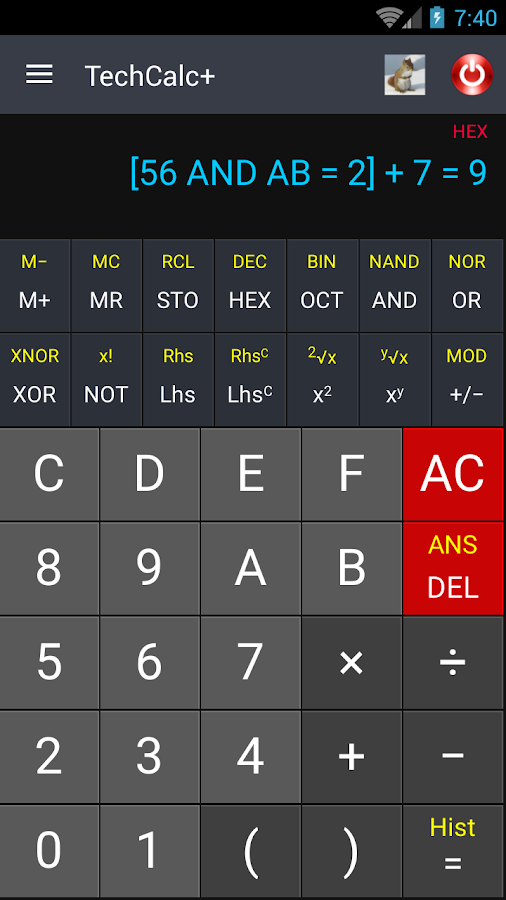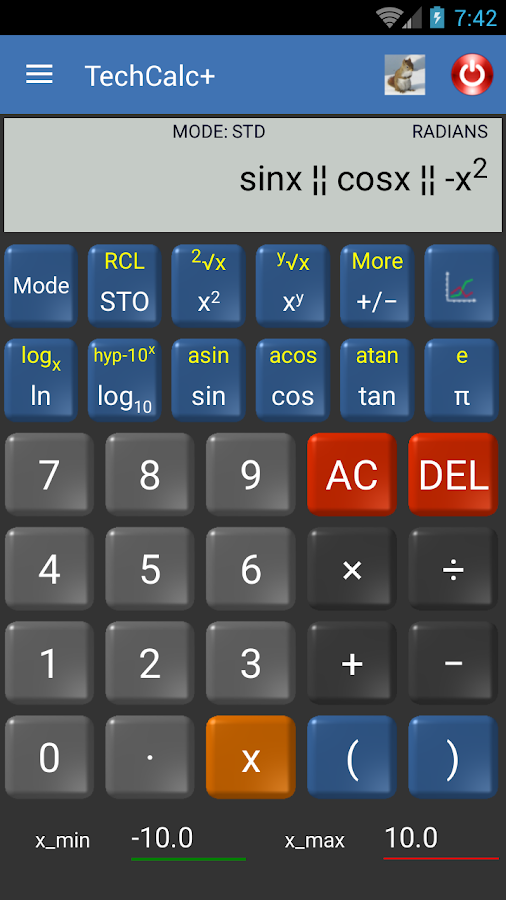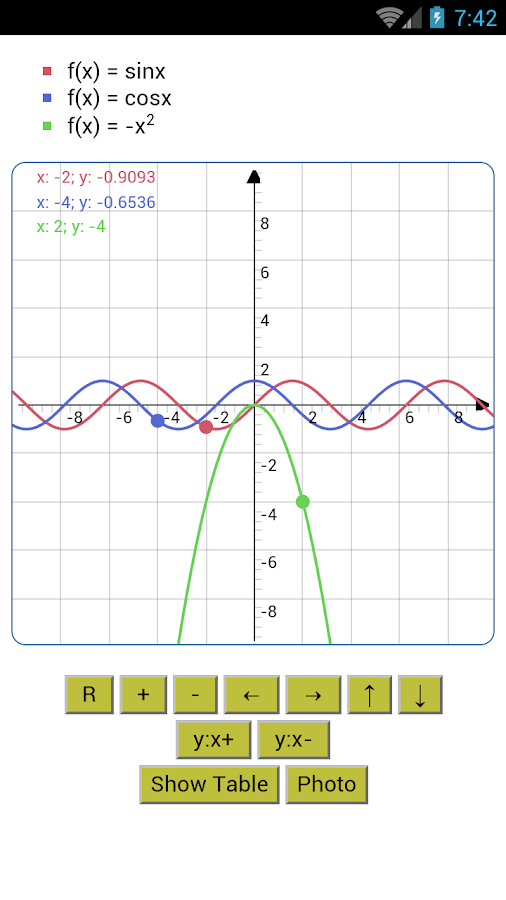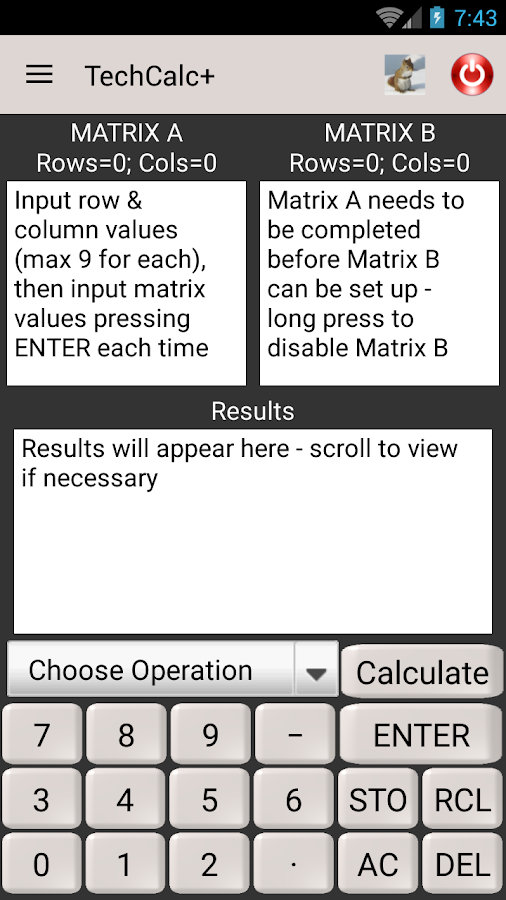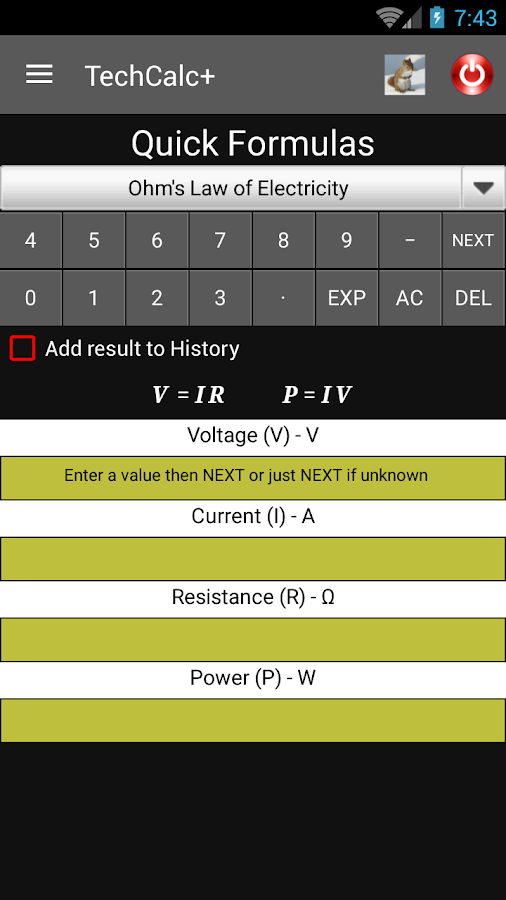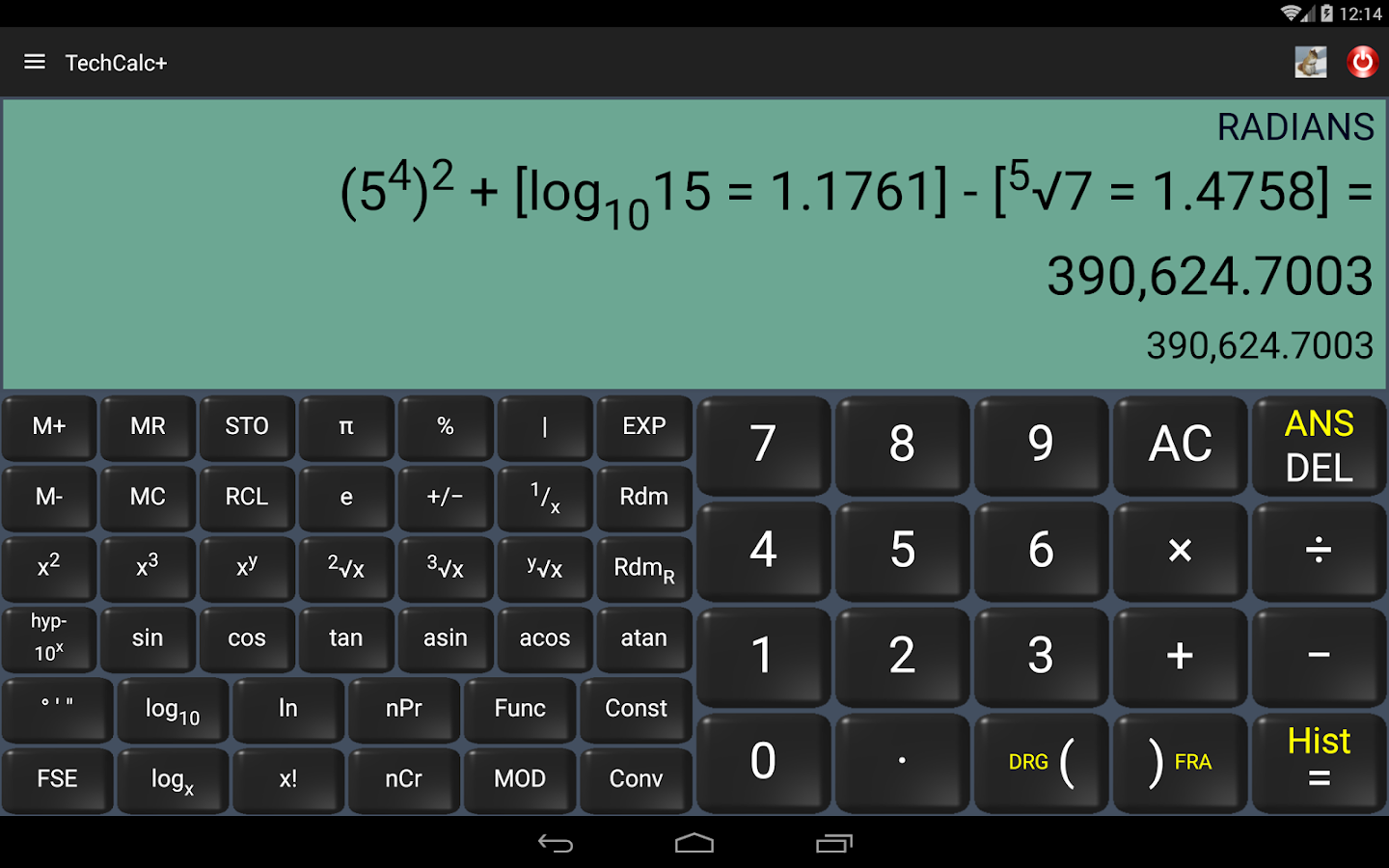# WHAT'S NEW

15/08 (ver 3.9.8)
* added new names of chemical elements 113, 115, 117 and 118 (the names are subject to a formal public review and, if there are no objections, they will be approved formally by the IUPAC Council on November 8, 2016)
* added Electric Current to Conversion Factors
* bug fixes

Requires Android-4.0 and up